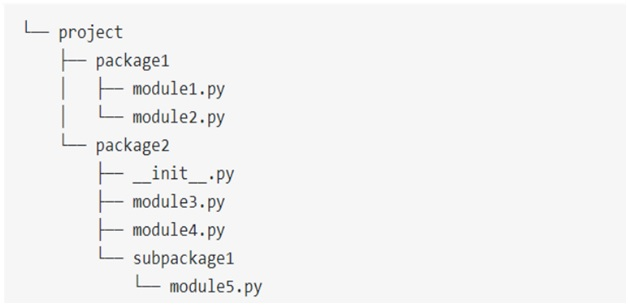# Absolute vs Relative Imports in Python

Here, we are going to learn what are absolute and relative imports in python, how they works?
Submitted by Akash Kumar, on November 16, 2017

A Python module is basically a file that contains ".py" extension and a Python package is any normal folder or directory that contains modules inside it.

How import works?

To understand how import basically works in python, Let us import a module named "abc" in python.

```    import abc
```

After seeing the above statement the first thing that Python will do is to look up the name XYZ in sys.modules. This is basically a cache of all modules that have been imported previously.

If this name is not found in the cache module then the python will proceed its searching through a list of the built-in module. This built-in module is basically pre-installed with python and can be seen through Python Standard Library. If the name still is not available at the built-in modules then the python will search for it in a list of directories that are basically defined by sys.path.

When Python finally finds the module it will bind it to a name in the local scope. This means that XYZ is now defined and it can be used in the current file without throwing an error called NameError.

If the name or file is still not found then we'll get an error called ModuleNotFoundError.

Syntax:

In python we can write import statement in two ways:

1. Import Resource Directly
Ex: import XYZ
2. Import Resource from another package or module
Ex: from xyz import abd

Styling of Basic Import statement

The following points everyone should keep in mind while writing the import statement:

1. These statements should always be written at the top of the file after any type of module comments.
2. These statements should be divided based on how it is imported,these are basically divided into three groups as follows:
• Standard library import
• Third-party import
• Local application imports

### 1) Absolute import

An absolute import basically specifies that the resource to be imported must use its full path from the project’s root folder.

For example:

Let us consider a project directory that has two subdirectory named package 1 and package 2. Where package 1 further contains two python file named module1.py and module2.py. And package 2 contains three .py files and a directory called subpackage1 which contain a .py file.Let’s assume the following:

package1 contains module2.py which contains a function named function2.

package2 contains __init__.py contains a class named class2.

package2 contains subpackage1 which basically contains module5.py which basically contains a function named function3.

Following are some of the practical example of absolute imports:

```    from package1 import module1
from package2.subpackage1.module5 import function3
```

Here we have basically given a detailed path for each package or file basically from the top-level package folder. This is basically similar to that file path, but here we have uses a dot (.) instead of a slash (/) that are used in the file path.

These imports are preferred because of the following advantages:

1. These imports are quite clear and straightforward.
2. These are the easiest way to tell where the imported resource is stored.
3. These statements remain same even if the current location of imported statement changes.

Because of these advantages PEP8 strongly recommends to use absolute import method.

But every coin has two faces absolute import method posses some disadvantage to these are as follows.

These imports can get quite verbose too based on the complexity of the structure of the directory. let's imagine a statement like as follows:

from package1.subpackage2.subpackage3.subpackage4.subpackage5.module5 import function7

This seems somewhat ridiculous.

### 2) Relative Imports

A relative import basically specifies that the resource that has been imported is relative to its current location—that is simply the location where the import statement is present.

Syntax:

Basically, the syntax of a relative import totally depends on the current location as well as the location where the module, package, or object is present which is needed to be imported.

Following are some of the examples of Relative Import:

```    from .module import sclass
from ..package import function
from . import sclass
```

Here a single dot(.) symbol basically refers to the current location or the same directory. Double dot(..) basically refers to the parent directory. And three dot(...) basically means that we are in grandparent directory.

One basic clear advantage of relative imports is that they are quite succinct. Based on the current location they can turn the ridiculously long import statement into something as simple as the following statement:

```    from ..subpackage4.module5 import function6
```

Relative Import too posses some disadvantages such as follows:

These imports can be quite messy basically for shared projects where directory structure is likely to change to every time. Relative imports are also not as readable or understandable like absolute ones, and these import statements are not that easy to tell the location of the imported resources.